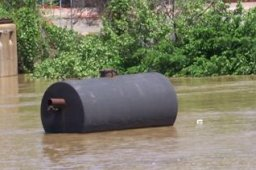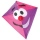# Floating barrel

Barrel (cylinder shape) floats on water, top of the barrel is 8 dm above water, and the width of surfaced barrel part is 23 dm. Barrel length is 24 dm.

Calculate the volume of the barrel.

V =  11343.3 dm3

### Step-by-step explanation:Did you find an error or inaccuracy? Feel free to write us. Thank you!Tips to related online calculators
Do you want to convert length units?
Tip: Our volume units converter will help you with the conversion of volume units.
Pythagorean theorem is the base for the right triangle calculator.

#### You need to know the following knowledge to solve this word math problem:

We encourage you to watch this tutorial video on this math problem:

## Related math problems and questions:

• Surface area of the topA cylinder is three times as high as it is wide. The length of the cylinder’s diagonal is 20 cm. Find the surface area of the top of the cylinder.
• A kiteChildren have a kite on an 80m long rope, which floats above a place 25m from the place where children stand. How high is the dragon floating above the terrain?
• The cylinder baseThe cylinder with a base of 8 dm2 has a volume of 120 liters. From a cylinder filled with water, 40 liters of water was removed. At what height from the bottom /with precision to dm/ is the water level?
• Body diagonalCalculate the cube volume, whose body diagonal size is 75 dm. Draw a picture and highlight the body diagonal.
• Right isoscelesCalculate area of the isosceles right triangle which perimeter is 26 cm.
• Cube diagonalsCalculate the length of the side and the diagonals of the cube with a volume of 27 cm3.
• Triangular prismThe triangular prism has a base in the shape of a right triangle, the legs of which is 9 cm and 40 cm long. The height of the prism is 20 cm. What is its volume cm3? And the surface cm2?
• Volume and surfaceCalculate the volume and surface area of the cylinder when the cylinder height and base diameter is in a ratio of 3:4 and the area of the cylinder jacket is 24 dm2.
• Find theFind the surface area of a regular quadrilateral pyramid which has a volume of 24 dm3 and a height of 45 cm.
• Reverse Pythagorean theoremGiven are lengths of the sides of the triangles. Decide which one is rectangular: Δ ABC: 77 dm, 85 dm, 36 dm ... Δ DEF: 55 dm, 82 dm, 61 dm ... Δ GHI: 24 mm, 25 mm, 7 mm ... Δ JKL: 32 dm, 51 dm, 82 dm ... Δ MNO: 51 dm, 45 dm,
• CylinderThe cylinder surface is 922 dm2. Its height is equal to the radius of the base. Calculate the height of this cylinder.
• SquareCalculate the area of the square shape of the isosceles triangle with the arms 50m and the base 60m. How many tiles are used to pave the square if the area of one tile is 25 dm2?
• Square vs rectangleSquare and rectangle have the same area contents. The length of the rectangle is 9 greater and width 6 less than side of the square. Calculate the side of a square.
• Cube surface and volumeFind the surface of the cube with a volume of 27 dm3.
• TentCalculate how many liters of air will fit in the tent that has a shield in the shape of an isosceles right triangle with legs r = 3 m long the height = 1.5 m and a side length d = 5 m.
• Diagonal 20Diagonal pathway for the rectangular town plaza whose length is 20 m longer than the width. if the pathway is 20 m shorter than twice the width. How long should the pathway be?
• Diameter = heightThe cylinder's surface, the height of which is equal to the diameter of the base, is 4239 cm square. Calculate the cylinder volume.